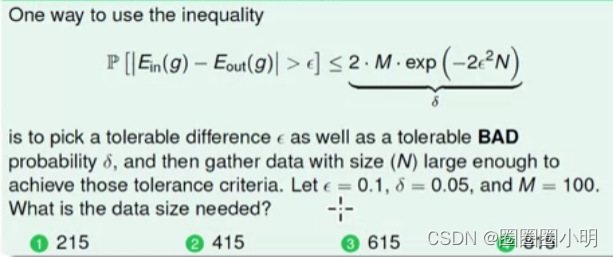# 【机器学习习题】估计一个模型在未见过的数据上的性能- P[|E_in(g) - E_out(g)| > ε]：这是泛化误差超过ε的概率
- ε：可容忍的差值。
- M：假设模型类别的大小（例如，假设我们正在训练的模型有多少个可能的候选）。
- N：数据集的大小。
- c：一个常数，通常设置为1。

P[|E_in(g) - E_out(g)| > ε] ≤ 2*M*exp(-2*ε^2*N)

2*M*exp(-2*ε^2*N) = δ

2*100*exp(-2*0.1^2*N) = 0.05

exp(-2*0.1^2*N) = 0.05 / (2*100)

exp(-2*0.01*N) = 0.00025

-2*0.01*N = ln(0.00025)

N = ln(0.00025) / (-2*0.01)

N ≈ 6907.76